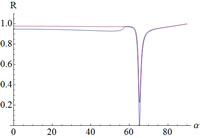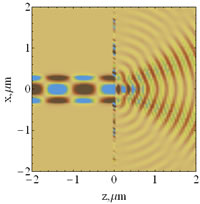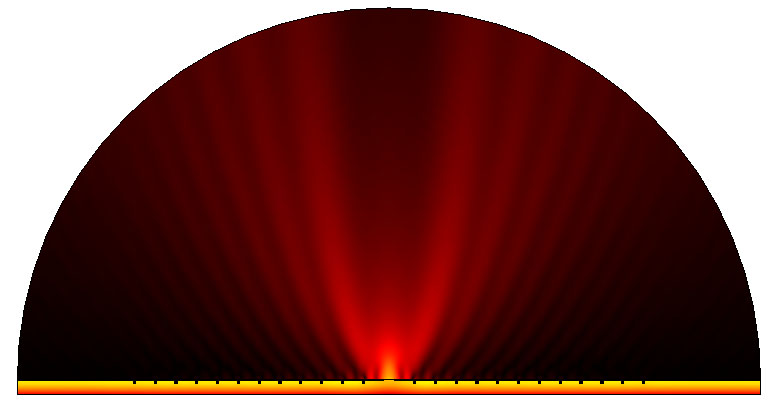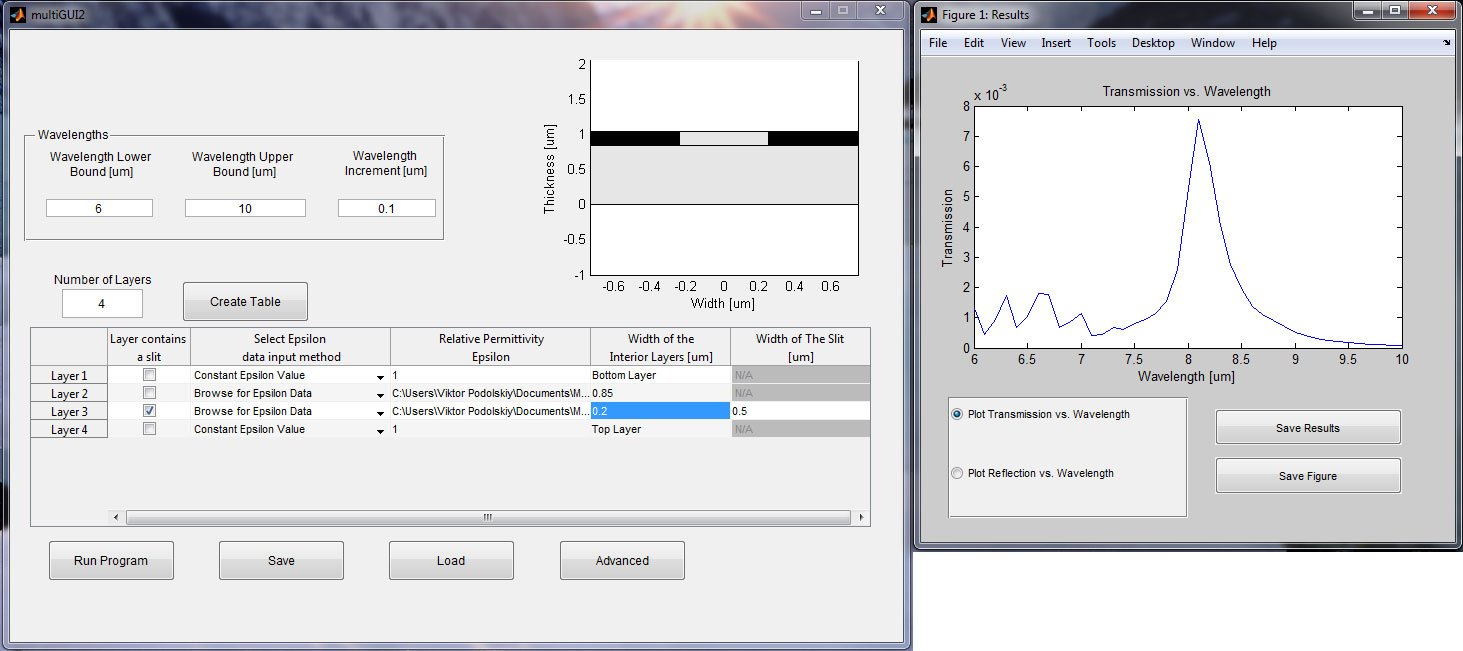Research Codes

Here you will find some of the codes developed and used in our group for applied electromagnetic projects. You are free to download and use these in your research or applications. Reference to this www or to original publication [see below] is requested.## 1D Transfer Matrix Approach

zip [reference the www]. The package contains Mathematica Module and an example of its use. The code can calculate Reflectance and Transmittance of an array of metal/dielectric/uniaxial layers, calculate dispersion of waveguide modes suppported by the multilayer systems, as well as to calculate field distribution in the layered structures.## 2D Transfer Matrix Formalism

[source codes; and sample data output ]; [reference J.Opt.Soc.Am B 26, B102 (2009)]. The package contains the implementation of the mode-matching formalism capable of calculating the field distribution in an array of planar waveguides; both open guides and closed guides are supported, and coupling between the system supporting different number of modes, yielding rectangular Transfer Matrices, is implemented.## Calculating mid-field profiles of plasmonic structures

[comsol geometry setup (58 Kb) and full comsol solution (70 Mb)]; [reference Optics Express, 19(10), 9269 (2011)].

The files, that can be opened in COMSOL Multiphysics 3.5 illustrate a typical geometry/meshing settings used for design of plasmonic beaming structures and for analysis of their performance.

The model does a parametric sweep over 7...14 wavelength range, and requires ~2Gb RAM to run. The smaller file contains the geometry and model settings; it needs to be run to generate meshes and solutions. The larger file contains solutions; Beaming wavelength is close to 9.5 microns## Calculating transmission through subwavelength slits

[matlab-based GUI (300 Kb); wavelength-dependent permittivity for doped semiconducting ENZ material (5 Kb)]];
[reference Phys.Rev.Lett.107, 133901 (2011)].

This compiled matlab code runs under MS Windows x64; it requires Matlab Compiler Runtime for Matlab R2012b (free download from http://www.mathworks.com/products/compiler/mcr/).

The code calculates transmission through thin slits in perfectly conducting screens for variety of materials. It can be also utilized to calculate transmission/reflection of arbitrary layered structure.

## RCWA codes

source codes ( zipped matlab).
A versatile implmentation of the Rigorous Coupled Wave Analysis, capable of calculating optical response of periodic materials with two-dimensional unit cell.

compiled GUI (exe). Windows only.

## Large-concentration nanowire effective medium codes

The ( zipped matlab) file contains matlab functions required to calculate effective medium properties of nanowire composites. It is assumed that the unit cell of the composite has rectangular shape, and the nanowire itself has (possibly-multishelled) cylindrical structure. The code relies on quasistatic approximation, but is not limited to the dipole approximation. Therefore, the unit cell must be subwavelength, but the concentration of nanowires inside the unit cell does not have to be small.
The attached functions calculate the field distributions, as well as effective medium parameters.